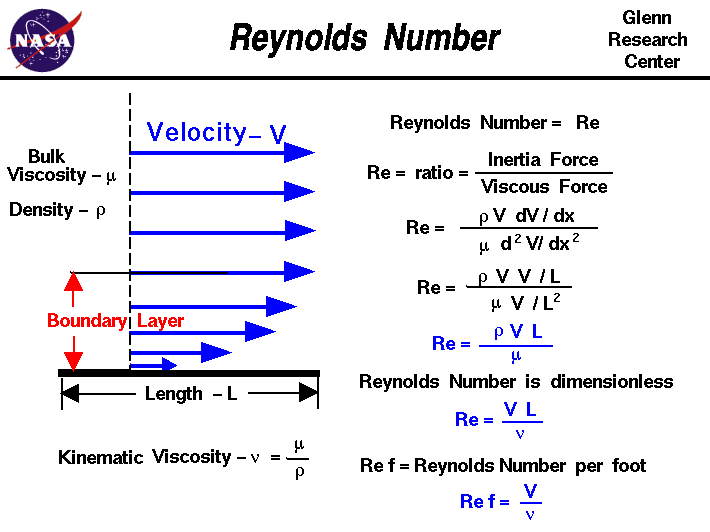+ Text Only Site
+ Non-Flash Version
+ Contact GlennAs an object moves through the atmosphere, the gas molecules of the atmosphere near the object are disturbed and move around the object. Aerodynamic forces are generated between the gas and the object. The magnitude of these forces depend on the shape of the object, the speed of the object, the mass of the gas going by the object and on two other important properties of the gas; the viscosity, or stickiness, of the gas and the compressibility, or springiness, of the gas. To properly model these effects, aerodynamicists use similarity parameters which are ratios of these effects to other forces present in the problem. If two experiments have the same values for the similarity parameters, then the relative importance of the forces are being correctly modeled. Representative values for the properties of air are given on another page, but the actual value of the parameter depends on the state of the gas and on the altitude. Aerodynamic forces depend in a complex way on the viscosity of the gas. As an object moves through a gas, the gas molecules stick to the surface. This creates a layer of air near the surface, called a boundary layer, which, in effect, changes the shape of the object. The flow of gas reacts to the edge of the boundary layer as if it was the physical surface of the object. To make things more confusing, the boundary layer may separate from the body and create an effective shape much different from the physical shape. And to make it even more confusing, the flow conditions in and near the boundary layer are often unsteady (changing in time). The boundary layer is very important in determining the drag of an object. To determine and predict these conditions, aerodynamicists rely on wind tunnel testing and very sophisticated computer analysis. The important similarity parameter for viscosity is the Reynolds number. The Reynolds number expresses the ratio of inertial (resistant to change or motion) forces to viscous (heavy and gluey) forces. From a detailed analysis of the momentum conservation equation, the inertial forces are characterized by the product of the density r times the velocity V times the gradient of the velocity dV/dx. The viscous forces are characterized by the dynamic viscosity coefficient mu times the second gradient of the velocity d^2V/dx^2. The Reynolds number Re then becomes: Re = (r * V * dV/dx) / (mu * d^2V/dx^2) The gradient of the velocity is proportional to the velocity divided by a length scale L. Similarly, the second derivative of the velocity is proportional to the velocity divided by the square of the length scale. Then: Re = (r * V * V/L) / (mu * V / L^2) Re = (r * V * L) / mu The Reynolds number is a dimensionless number. High values of the parameter (on the order of 10 million) indicate that viscous forces are small and the flow is essentially inviscid. The Euler equations can then be used to model the flow. Low values of the parameter (on the order of 1 hundred) indicate that viscous forces must be considered. The Reynolds number can be further simplified if we use the kinematic viscosity nu that is euqal to the dynamic viscosity divided by the density: nu = mu / r Re = V * L / nu Here's a Java program to calculate the coefficient of viscosity and the Reynolds number for different altitude, length, and speed. This page shows an interactive Java applet which calculates the viscosity coefficient and the Reynolds number for an input velocity, length, and altitude. To change input values, click on the input box (black on white), backspace over the input value, type in your new value, and hit the Enter key on the keyboard (this sends your new value to the program). You will see the output boxes (yellow on black) change value. You can use either Imperial or Metric units and you can input either the Mach number or the speed by using the menu buttons. Just click on the menu button and click on your selection. The non-dimensional Mach number and Reynolds number are displayed in white on blue boxes. If you are an experienced user of this calculator, you can use a sleek version of the program which loads faster on your computer and does not include these instructions. You can also download your own copy of the program to run off-line by clicking on this button: The Similarity Parameter Calculator was modified in May, 2009, by Anthony Vila, a student at Vanderbilt University, during a summer intern session at NASA Glenn. For some problems we can divide the Reynolds by the length scale to obtain the Reynolds number per foot Ref. This is given by: Ref = V / nu The Reynolds number per foot (or per meter) is obviously not a non-dimensional number like the Reynolds number. You can determine the Reynolds number per foot using the calculator by specifycing the length scale to be 1 foot. Activities: Guided Tours Navigation ..Beginner's Guide Home Page+ Inspector General Hotline + Equal Employment Opportunity Data Posted Pursuant to the No Fear Act + Budgets, Strategic Plans and Accountability Reports + Freedom of Information Act + The President's Management Agenda + NASA Privacy Statement, Disclaimer, and Accessibility CertificationEditor: Tom Benson NASA Official: Tom Benson Last Updated: May 07 2021 + Contact Glenn